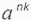# GATE | GATE CS 2011 | Question 42

Definition of a language L with alphabet {a} is given as following.

`             L={| k>0, and n is a positive integer constant}`

What is the minimum number of states needed in DFA to recognize L?
(A) k+1
(B) n+1
(C) 2^(n+1)
(D) 2^(k+1)